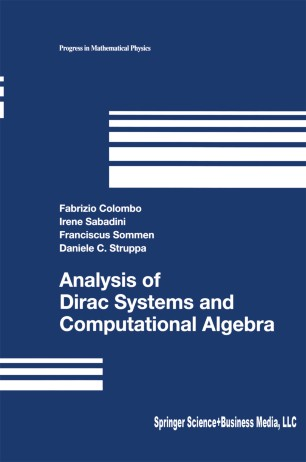# Analysis of Dirac Systems and Computational AlgebraTextbook

Part of the Progress in Mathematical Physics book series (PMP, volume 39)

1. Front Matter
Pages i-xv
2. Fabrizio Colombo, Irene Sabadini, Franciscus Sommen, Daniele C. Struppa
Pages 1-91
3. Fabrizio Colombo, Irene Sabadini, Franciscus Sommen, Daniele C. Struppa
Pages 93-138
4. Fabrizio Colombo, Irene Sabadini, Franciscus Sommen, Daniele C. Struppa
Pages 139-207
5. Fabrizio Colombo, Irene Sabadini, Franciscus Sommen, Daniele C. Struppa
Pages 209-266
6. Fabrizio Colombo, Irene Sabadini, Franciscus Sommen, Daniele C. Struppa
Pages 267-306
7. Fabrizio Colombo, Irene Sabadini, Franciscus Sommen, Daniele C. Struppa
Pages 307-311
8. Back Matter
Pages 313-332

### Introduction

The subject of Clifford algebras has become an increasingly rich area of research with a significant number of important applications not only to mathematical physics but to numerical analysis, harmonic analysis, and computer science.

The main treatment is devoted to the analysis of systems of linear partial differential equations with constant coefficients, focusing attention on null solutions of Dirac systems. In addition to their usual significance in physics, such solutions are important mathematically as an extension of the function theory of several complex variables. The term "computational" in the title emphasizes two main features of the book, namely, the heuristic use of computers to discover results in some particular cases, and the application of Gröbner bases as a primary theoretical tool.

Knowledge from different fields of mathematics such as commutative algebra, Gröbner bases, sheaf theory, cohomology, topological vector spaces, and generalized functions (distributions and hyperfunctions) is required of the reader. However, all the necessary classical material is initially presented.

The book may be used by graduate students and researchers interested in (hyper)complex analysis, Clifford analysis, systems of partial differential equations with constant coefficients, and mathematical physics.

### Keywords

Algebra Category theory Cohomology Complex Analysis Differential Equations Numerical & Computational Tech Quantum Field Theory mathematical physics partial differential equation

#### Authors and affiliations

1. 1.Dipartimento di MatematicaPolitecnico di MilanoMilanoItaly
2. 2.Faculty of Engineering Department of Mathematical AnalysisGhent UniversityGhentBelgium
3. 3.Department of Mathematical SciencesGeorge Mason UniversityFairfaxUSA

### Bibliographic information

• Book Title Analysis of Dirac Systems and Computational Algebra
• Authors Fabrizio Colombo
Franciscus Sommen
Daniele C. Struppa
• Series Title Progress in Mathematical Physics
• DOI https://doi.org/10.1007/978-0-8176-8166-1
• Publisher Name Birkhäuser, Boston, MA
• eBook Packages
• Hardcover ISBN 978-0-8176-4255-6
• Softcover ISBN 978-1-4612-6469-9
• eBook ISBN 978-0-8176-8166-1
• Edition Number 1
• Number of Pages XV, 332
• Number of Illustrations 0 b/w illustrations, 0 illustrations in colour
• Topics
• Buy this book on publisher's site

## Reviews

From the reviews:

"The book presents a uniform treatment of some fundamental differential equations for physics. Maxwell and Dirac equations are particular examples that fall into this study. The authors concentrate on systems of linear partial differential equations with constatn coefficients n the Clifford algebra setting...The material is presented in a very accessible format...The book ends with a list of open problems that pertain to the topic." ---Internationale Mathematische Nachrichtén, Nr. 201

"The first 138 pages of this book are a good introduction to algebraic analysis (in the sense of Sato), and some computational aspects, in the setting of quaternionic analysis. But the core of the book is the study of different important systems of partial differential equations in the setting of Clifford analysis...The last chapter states some open problems and avenues of further research. A rich list of references, an alphabetic index and a list of notation close the volume. Well-written and with many explicit results, the book is interesting and is addressed to Ph.D. students and researchers interested in this field." ---Revue Roumaine de Mathématiques Pures et Appliquées

“Altogether the book is a pioneering, and quite successful, attempt to apply computational and algebraic techniques to several branches of hypercomplex analysis … The book provides a very different way to look at some important questions which arise when one tries to develop multi-dimensional theories.”(MATHEMATICAL REVIEWS)